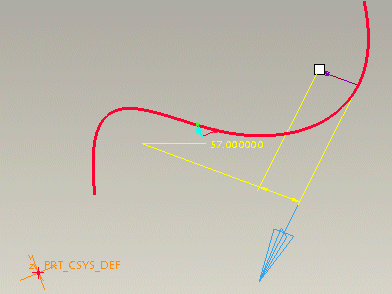Point Information on the Edge or Curve
A normal to the surface at a selected point is a normal curvature vector. Principal curvature vectors are determined as follows: for every plane that contains the normal vector, there is a curve of intersection of this plane with the surface. Creo Parametric evaluates all the intersection curves to determine the maximum and minimum curvature value.
There can be only one curvature value if it is the same for all planes. The curvature values are called the principal curvatures of the surface at the specified point.
Curvatures can be positive or negative. If the curve turns in the direction away from the normal, it is indicated by a negative sign. The intersection of planes that contain the principal curvatures with the tangent plane defines the principal directions.